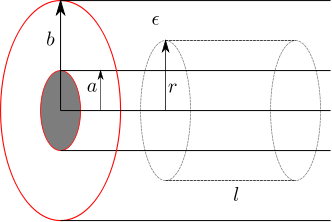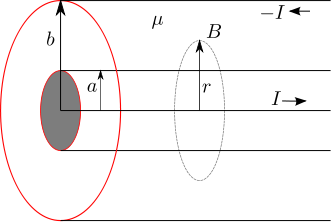- DSP log - http://www.dsplog.com -

GATE-2012 ECE Q16 (electromagnetics)

Posted By Krishna Sankar On November 25, 2012 @ 7:59 pm In GATE | No Comments

Question 16 on electromagnetics from GATE (Graduate Aptitude Test in Engineering) 2012 Electronics and Communication Engineering paper.

(D)Solution

To answer this question, am referring to the discussion on capacitance per unit length (Section 1.9) , inductance per unit length (Section 2.4) and characteristic impedance (Section 5.2) of a coaxial cable from  Fields and Waves in Communication Electronics, Simon Ramo, John R. Whinnery, Theodore Van Duzer (buy from Amazon.combuy from Flipkart.com ).

Finding the capacitance per unit length:

Using Gauss law  :

For a closed Gaussian surface , the electrical flux  is given by :

, where

is the electrical flux,

is the electric field,

is the vector representing the infinitesimal area on the surface ,

is the net charge enclosed by the surface and

is the permittivity of vacuum.

Let us use this result to find the electrical field in a coaxial cable formed by two conducting cylinders of radii and respectively with a dielectric between them (shown in the figure below).Figure : Coaxial cable showing the imaginary Gaussian surface (cylinder with radius and length )

For finding the electric field in region , assume a cylindrical Gaussian surface of radius and length (as shown by the dashed line).

Applying Gauss law,

,

where

is the charge per unit length,

total charge enclosed is the charge per unit length multiplied by the length i.e. and

is the permittivity ( is the permittivity of vacuum, is the permittivity of the material).

Given that the electrical field is is parallel to the surface and is uniform across the Gaussian surface, it can be moved out of the integral, i.e

.

The term is the surface area of the cylinder with radius of length and is,

.

Substituting, the electric field in the region is,

.

With the above electric field, the potential difference between the coaxial cylinders is computed as,

.

The capacitance between two electrodes is defined as the charge  on each electrode per volt of potential difference between then,

.

Assuming that the field is only radial and the total charge per unit length is , the capacitance per unit length is

.

Finding the inductance per unit length:

Using Ampere’s Law  :

The total current inside a closed curve  is the line integral of the magnetic field (in Tesla)

,

where

is the magnetic field (in Tesla)

is the vector representing the infinitesimal line on the closed loop ,

is the net current enclosed by the closed loop and

is the permeability of vacuum .

Let us use this result to find the magnetic field in a coaxial cable formed by two conducting cylinders of radii and respectively with a dielectric having permeability of between them (shown in the figure below).Figure : Coaxial cable showing the imaginary Amperian loop  (circle with radius )

Applying Ampere’s law,

,

where

is the permeability ( is the permeability of vacuum. is the permeability of the material).

Given that the magnetic field is  is parallel to the line and is uniform across the closed loop, it can be moved out of the integral, i.e

.

The term is the circumference of the circle with radius and is, .

Substituting, the magnetic field in the region is,

.

The inductance is defined as ratio of magnetic field over a surface and the the current.

.

For a unit length, the integral of magnetic field in the region is,

.

Substituting, the inductance per unit length is,

.

Note :

YouTube videos uploaded by user lasseviren1  aided me in understanding the  integrals in electric field and magnetic field. Do checkout the play-lists : Gauss’s Law  , Sources of Magnetic Fields 

The characteristic impedance :

From wiki entry on characteristic impedance , the general expression for the characteristic impedance of a transmission line is

,

where,

is the resistance per unit length,

is the inductance per unit length,

is the capacitance per unit length,

is the conductance per unit length and

is the angular frequency.

Assuming a loss less transmission line, i.e. , the equation reduces to,

.

Substituting the expressions for inductance per unit length and capacitance per unit length for a coaxial cable,

.

Substituting the numbers from the problem at hand,

,

the characteristic impedance is,

.

The calculated choice is not listed in the options. However if we ignore the term, the calculated number comes to around . That does not help, does it?References

 GATE Examination Question Papers [Previous Years] from Indian Institute of Technology, Madras http://gate.iitm.ac.in/gateqps/2012/ec.pdf 

 Fields and Waves in Communication Electronics, Simon Ramo, John R. Whinnery, Theodore Van Duzer (buy from Amazon.combuy from Flipkart.com ).

 The youtube videos uploaded by user lasseviren1  aided me in understanding the  integrals in electric field and magnetic field. Do checkout the play-lists : Gauss’s Law 

Sources of Magnetic Fields 

 Characteristic impedance 

 Ampere’s Law 

 Gauss law 

  Permeability 

 Permittivity 

 Electrical Flux 

URL to article: http://www.dsplog.com/2012/11/25/gate-2012-ece-q16-electromagnetics/

URLs in this post:

 Gauss law: http://en.wikipedia.org/wiki/Gauss%27s_law

 electrical flux: http://en.wikipedia.org/wiki/Electric_flux

 permittivity : http://en.wikipedia.org/wiki/Permittivity

 Ampere’s Law: http://en.wikipedia.org/wiki/Amp%C3%A8re%27s_circuital_law

 permeability of vacuum: http://en.wikipedia.org/wiki/Vacuum_permeability